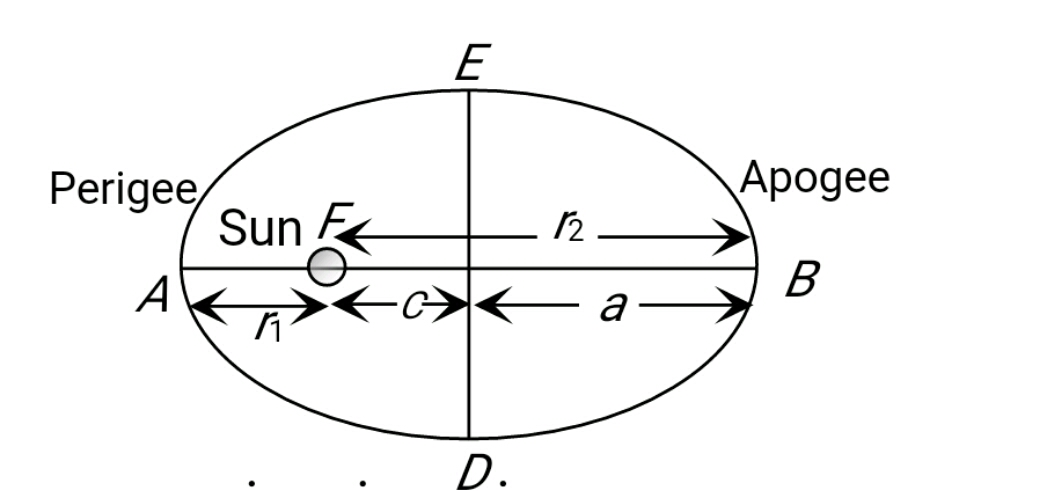Q

# If a planet orbiting around sun,then what is the Velocity of a Planet at Apogee and Perigee ???

Views

For the below figureIf Eccentricity is given by

(e) =

Then the velocity of the planet at Apogee and Perigee in terms of Eccentricity is given by

The velocity of the planet at apogee

=The velocity of the planet at apogee

Exams
Articles
Questions